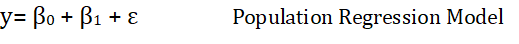# Simple Linear Regression Prerequisite

## Least Square MethodLets understand how the coefficients of regression are calculated using the least square model. In simple regression analysis, one one regressor is involved but here there are multiple regressors, we call it multiple regression analysis. Using least square estimation method, regression coefficients can be estimated by minimizing the sum of squares of difference between the observed value and the straight line using the minimum variance concept.  The below derivation is for a simple linear equation with multiple observations. The sample regression model denotes the letter i as subscript used in summation for integers 1,2 ..n depending on the number of observations. The sample regression model is different from population regression model with the introduction of i as a subscript.

###### Above fig Simple Linear Equation Diagram | The middle RoadUsing sample regression model,on the left. i donates the number of observations; i= 1,2,3 …………. nUsing least square estimation method, regression coefficients can be estimated by minimizing the sum of squares of difference between the observed value and the straight line using the minimum variance concept. The equation on the right will be differentiated with respect to the two coefficients and the first order of the differential equated to zero. The differentiation would be done taking one coefficient parameter at a time. The below example is from the book Introduction to Linear Regression Analysis by Douglas Montgomery,  Elizabeth Peck, G. Geoffrey Vining. However, the complete derivation is solved by The middle Road and written below.Using the above equation 1, we can find the fitted values of both the coefficients of regression. The fitted values of the coefficients of regression are donated with a cap in the equation. The values of Y (Dependent Variable or Response Variable) and X, the Independent/Predictor Variable or Regressor are the averages for the observations of variables in the sample regression model.

#### Refer to the side equations.

Now solving for the other regression coefficient, differentiating and equating the equation to zero.#### The derivation does not include summation sign for the Beta1 to making the analysis more simple. The summations are included at the end of the equation.